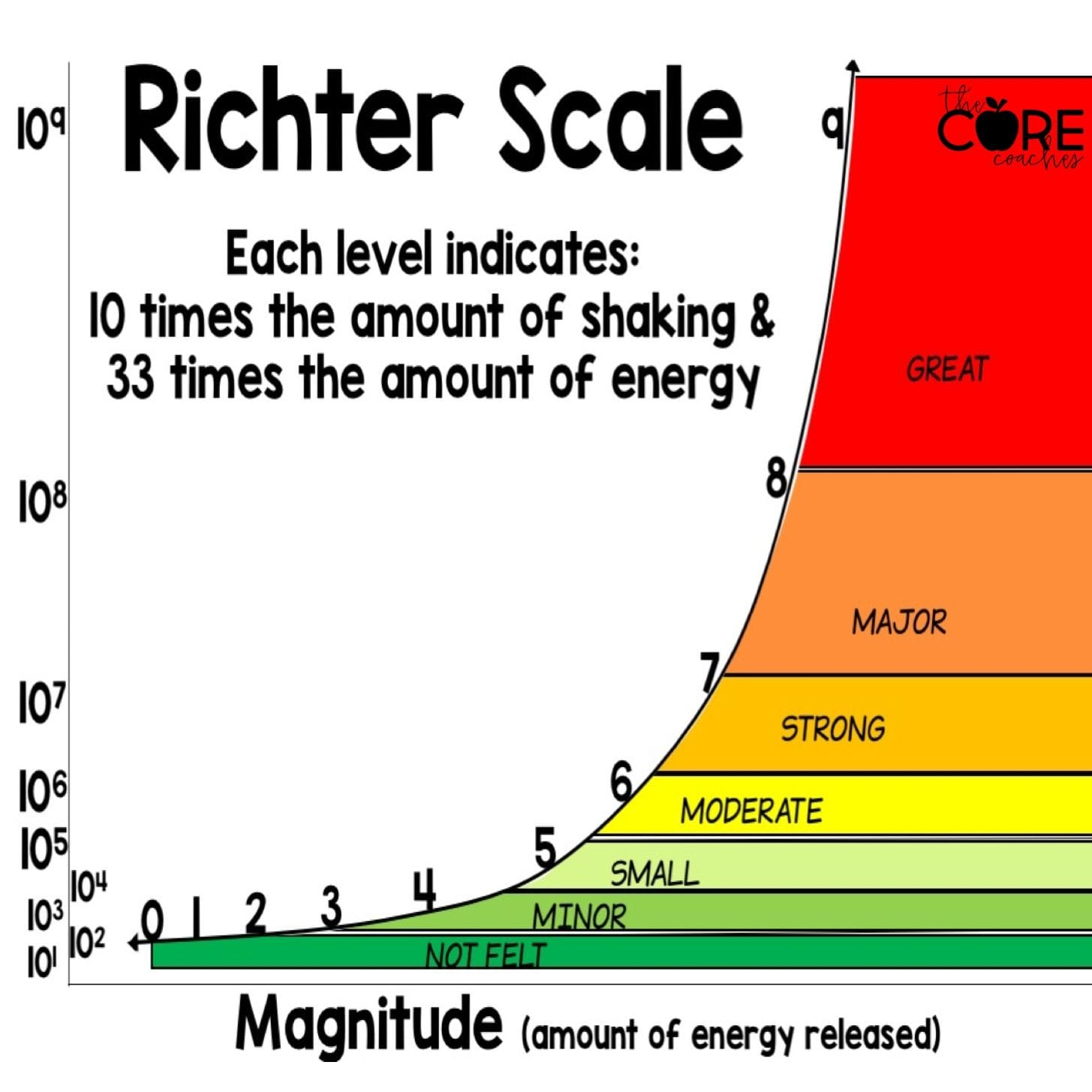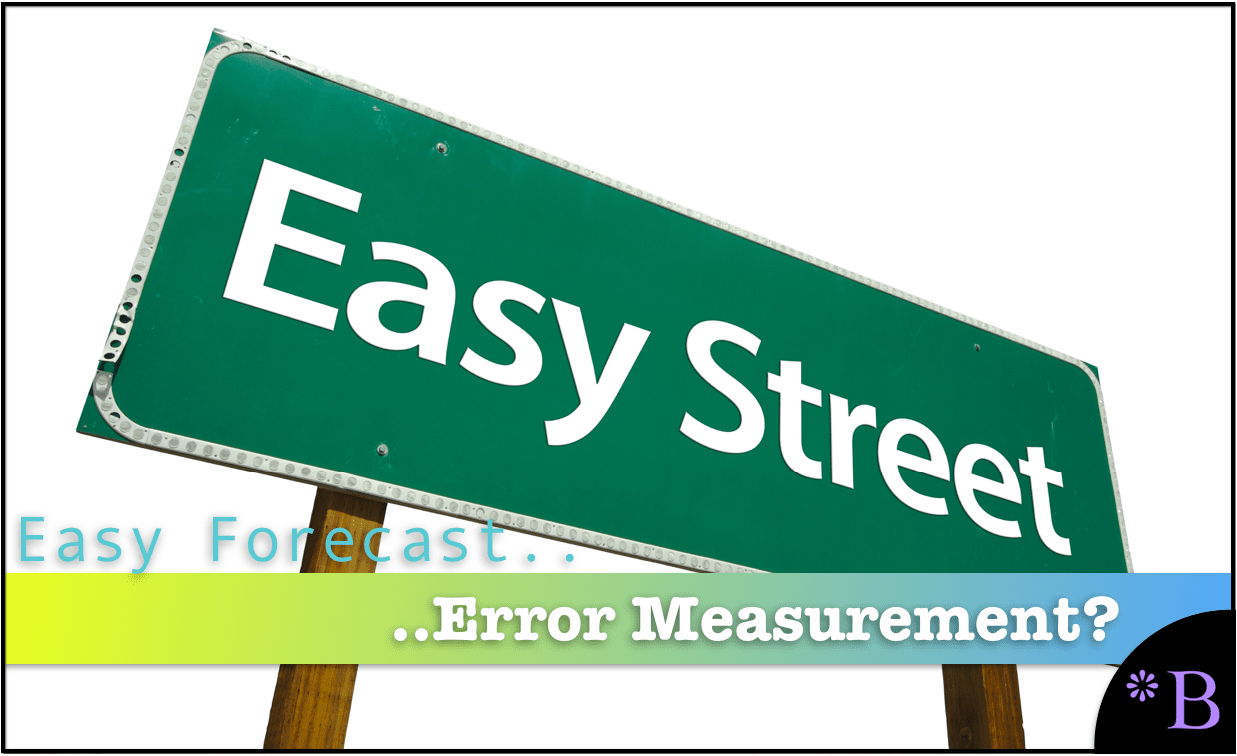# How sMAPE is Calculated for Forecast Error Measurement

## Executive Summary

• sMAPE is one of the alternatives presented for limitations with MAPE forecast error measurement.
• sMAPE is generally low in effectiveness in providing feedback to improve the forecast.## Text Introduction (Skip if You Watched the Video)

sMAPE, or Symmetrical Mean Absolute Percentage Error, is one of the significant but uncommon forecast error measurements. However, its complexity in calculation and difficulty in explanation makes it a distant third to the far more common MAD and MAPE forecast error calculations. A major problem with sMAPE is that it does not improve the ability to improve forecast accuracy. At Brightwork, we do not use sMAPE when we perform forecast analysis. In this article, you will learn about the issues with sMAPE.

If you want to see our references for this article and other related Brightwork articles, see this link.

## How sMAPE is Calculated

How sMAPE is calculated not that common of a question due to the fact sMAPE is infrequently used.

How sMAPE is calculated is as follows.

1. Take the absolute forecast minus the actual for each period that is being measured.
2. Square the result
3. Obtain the square root of the previous result.

The formula is..

## = Square Root(Squared(F – A))

Like MAD and RMSE, sMAPE uses squared values, and sMAPE is more complicated to calculate than either MAD or RMSE.

Because how sMAPE is calculated is that it is squared, the error is not proportional — meaning that more substantial errors become much more substantial as they are squared. Any error measurement that is not proportional makes it intuitively more difficult to understand than a proportional measure. How is, particularly, a non-forecasting focused person supposed to interpret nonproportional forecast errors? Our brain is designed to understand proportionality.For example, the Richter Scale is a logarithmic scale, which allows a relatively small number of values to represent large magnitudes in difference. However, it also means that humans cannot calculate the difference between different earthquake values.

## The Same Problem With All the Standard Forecast Error Measurements

Due to its lack of proportionality and high complexity in the calculation, sMAPE was never a forecast error method that we used before developing our own. But I did test it and found it not useful. However, in addition to this lack of proportionality, sMAPE shares the same problems with all standard forecast error measurements.

These include the following:

1. They are difficult to weigh. And any forecast error aggregated beyond the product location combination must be weighed to make any sense.
2. They make comparisons between different forecasting methods overly complicated.
3. They lack the context of the volume of the demand history or the price of the product being forecasted, meaning that the forecast errors must be provided with context through another formula.
4. They are difficult to explain, making them less intuitive than a different approach.

## Rob Hyndman Has the Following to Say on sMAPE

If all data and forecasts are non-negative, then the same values are obtained from all three definitions of sMAPE. But more generally, the last definition above from Chen and Yang is clearly the most sensible, if the sMAPE is to be used at all. In the M3 competition, all data were positive, but some forecasts were negative, so the differences are important. However, I can’t match the published results for any definition of sMAPE, so I’m not sure how the calculations were actually done.

Personally, I would much prefer that either the original MAPE be used (when it makes sense), or the mean absolute scaled error (MASE) be used instead. There seems little point using the sMAPE except that it makes it easy to compare the performance of a new forecasting algorithm against the published M3 results. But even there, it is not necessary, as the forecasts submitted to the M3 competition are all available in the Mcomp package for R, so a comparison can easily be made using whatever measure you prefer.

Something important here is that while it may be easy to compare performance for those highly mathematical individuals who compete in the M3/M4/M5 competition, it does not follow that it is easy for forecasting departments to use.

## Why Do the Standard Forecast Error Calculations Make Forecast Improvement So Complicated and Difficult?

It is important to understand forecasting error, but the problem is that the standard forecast error calculation methods do not provide this good understanding. In part, they don't let tell companies that forecast how to make improvements. If the standard forecast measurement calculations did, it would be far more straightforward and companies would have a far easier time performing forecast error measurement calculation.

## What the Forecast Error Calculation and System Should Be Able to Do

One would be able to for example:

1. Measure forecast error
2. Compare forecast error (For all the forecasts at the company)
3. To sort the product location combinations based on which product locations lost or gained forecast accuracy from other forecasts.
4. To be able to measure any forecast against the baseline statistical forecast.
5. To weigh the forecast error (so progress for the overall product database can be tracked)

##Getting to a Better Forecast Error Measurement Capability

A primary reason these things can not be accomplished with the standard forecast error measurements is that they are unnecessarily complicated, and forecasting applications that companies buy are focused on generating forecasts, not on measuring forecast error outside of one product location combination at a time. After observing ineffective and non-comparative forecast error measurements at so many companies, we developed, in part, a purpose-built forecast error application called the Brightwork Explorer to meet these requirements.

Few companies will ever use our Brightwork Explorer or have us use it for them. However, the lessons from the approach followed in requirements development for forecast error measurement are important for anyone who wants to improve forecast accuracy.